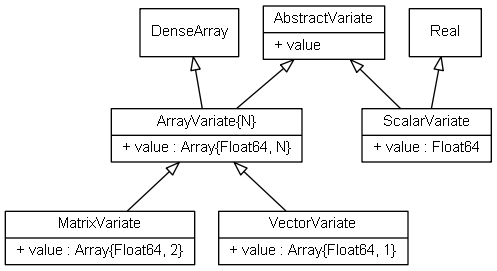# Variate¶

`ScalarVariate` and `ArrayVariate{N}` are abstract types that serve as the basis for several concrete types in the Mamba package. Conceptually, they represent data structures that store numeric values simulated from target distributions. Being abstract, these variate types cannot be instantiated and cannot have fields. They can, however, have method functions, which descendant subtypes will inherit. Such inheritance allows one to endow a core set of functionality to all subtypes by simply defining method functions once on the abstract types (see julia Types). Accordingly, a core set of functionality is defined for the variate types through the field and method functions discussed below. Although the (abstract) types do not have fields, their method functions assume that all subtypes will be declared with a `value` field.

## Declarations¶

```abstract type ScalarVariate <: Real
abstract type ArrayVariate{N} <: DenseArray{Float64, N}

const AbstractVariate = Union{ScalarVariate, ArrayVariate}
const VectorVariate = ArrayVariate{1}
const MatrixVariate = ArrayVariate{2}
```

## Type Hierarchy¶

Subtypes of the variate types include the Dependent, Logical, Stochastic, and SamplerVariate types.UML relational diagram of `Variate` types and their fields.

## Field¶

• `value::T` : scalar or array of `Float64` values that represent simulated values from a target distribution.

## Methods¶

Methods for `ScalarVariate` and `ArrayVariate` include mathematical operators, mathematical functions, and statistics defined in the base julia language for parent types `Real` and `DenseArray`. In addition, the following functions are provided.

Function Description
`logit(x)` log-odds
`invlogit(x)` inverse log-odds